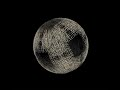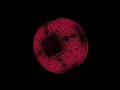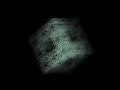Three ways to make 3D

Posted in: opengl , webgl , javascript , scala , processing , spde
Last week I got the chance to put my hands on some technologies I've been interested for some time now. I've created a basic 3D example of some fractals, and implemented it in 2D Canvas, WebGL and SPDE (Scala + Processing). The main goal of these experiments was to warm up a little bit in 3D, to learn WebGL, Scala and Processing. I had previous experience with 3D and OpenGL while developing V8-GL, an OpenGL ES port to V8, and also while developing the House of Cards visualization in OCaml. But these were other times. Now OCaml is dead, and there's a V8-GL port in the browser, which is called WebGL. I'm kind of proud to have chosen things that became sort of mainstream later on though: functional programming for graphics and hardware accelerated graphics with JavaScript :) .

2D Canvas

The 2D Canvas API has a limited capability for 3D graphics. In order to make 3D with it you can use some high level 3D engine like pre3d or three.js which provide classes to do mathy stuff in 3D and then use tricks like the pinhole camera model to project them on 2D. What's interesting about three.js is that it also provides SVG and WebGL renderers. These are awesome tools to make 3D, but since my example was more in the spirit of the JS1k demos I decided to start from scratch. The only thing I used was the Vector3 and Matrix4 classes from three.js. The result is quite interesting although it's CPU intensive. You can try it here if you have a 2D canvas enabled browser.To make this example I created a Shape object, that would create a list of particles placed in some particular shape:
var Shape = {
Sphere: function(objs, opts) {
var s = opts.s || 200,
atan2 = Math.atan2,
cos = Math.cos,
acos = Math.acos,
sin = Math.sin,
sqrt = Math.sqrt;

for(var i=0, l=objs.length; i&lt;l; i++) {
var obj = objs[i],
pos = obj.pos,
x = pos.x,
y = pos.y,
z = pos.z,
r = x * x + y * y + z * z,
theta = acos(z / sqrt(r)),
phi = atan2(y, (x || 1));

if(!obj.sphere) {
obj.sphere = new THREE.Vector4();
}
var pos = obj.pos, sphere = obj.sphere;
pos.x = sphere.x = s * sin(theta) * cos(phi);
pos.y = sphere.y = s * sin(theta) * sin(phi);
pos.z = sphere.z = s * cos(theta);
}
}
//...other shapes here...
};
I also created a tween object from scratch, which would provide transition equations (linear, Elastic, Bounce) for the particles movement. There are many tweeners around, but since I'm a big fan of MooTools and the way they use object mutability and functions as objects to create their tweener I implemented something in that spirit. The transition object code provides an object with methods (Trans.Elastic(delta), Trans.Bounce(delta)) and "submethods" as properties of these methods (Trans.Elastic.easeOut(delta), Trans.Bounce.easeInOut(delta)). That's the approach I like and I don't think it's that easy to emulate in other languages... Will Scala be up to the task? Here's some code for that transitions object:
//transitions object. can be used like
//Trans.linear, or Trans.Elastic.easeOut, etc.
var Trans = {
linear: function(p){
return p;
}
};

(function(){

//of the transition object/function
//i.e add easeIn/Out to the Elastic/Sine objects
var makeTrans = function(transition, params){
var trans = {
easeIn: function(pos){
return transition(pos, params);
},
easeOut: function(pos){
return 1 - transition(1 - pos, params);
},
easeInOut: function(pos){
return (pos <= 0.5)? transition(2 * pos, params) / 2
: (2 - transition(2 * (1 - pos), params)) / 2;
}
};
for(var p in trans) {
transition[p] = trans[p];
}
return transition;
};

//transition equations
var transitions = {

Sine: function(p){
return 1 - Math.sin((1 - p) * Math.PI / 2);
},

Elastic: function(p, x){
return Math.pow(2, 10 * --p)
* Math.cos(20 * p * Math.PI * (x || 1) / 3);
}
//...other transitions here...
};

//enhance the Trans object with new transitons
for(var p in transitions) {
Trans[p] = makeTrans(transitions[p]);
}

})();
Finally the main.js file contains init, loop and nextShape functions. The init function will call the Shape object methods, pre-calculating the particles positions, and will set an array with the shapes to iterate on. Loop will render each point to the canvas, by using the x and y coordinates of the particle. The z coordinate will determine the alpha color and radius of the particle. Once a fixed number of loops are triggered nextShape will be called to make the transition to the next shape. While this is a simple approach, 3D in the 2D canvas environment is quite limited, and very CPU intensive.

WebGL

Since most of the code used in the previous example was dealing with 3D objects and then projected into 2D space, this code can also be used when working with WebGL. Getting started with WebGL is much harder than with 2D Canvas though. First, there's all that setup of creating a program, compiling the shaders (which of course is GLSL code and not JavaScript itself), linking them into the program, initializing buffers, etc. Moreover, since there's not a fixed pipeline all that push/pop matrix stack has to be hand-coded now, which makes it quite difficult to create things without a framework. However, since this is a very simple example I used exactly the same things here than in the previous example. Here's the live example that you can see in a WebGL enabled browser (click here to get a WebGL enabled browser). Or you can check the video I made of the demo below (click here if you can't see the video): The rendering part is quite different though. You need to set all the vertex information into a buffer and send that data into the shaders. The shaders will get the data in attributes (which is per vertex information) or uniforms (data which will remain the same for all vertices). For example, all particles are colored the same, but each particle has a different position, so the code would look like this:
//draw scene
gl.viewport(0, 0, gl.viewportWidth, gl.viewportHeight);
gl.clear(gl.COLOR_BUFFER_BIT | gl.DEPTH_BUFFER_BIT);
perspective(45, gl.viewportWidth / gl.viewportHeight, 0.1, 500.0);
mvTranslate([0.0, 0.0, -150.0]);
mvRotateX(rx);
mvRotateY(ry);
for(var i=0, l=parts.length, vertices=[]; i&lt;l; i++) {
var p = parts[i].pos;
vertices.push(p.x, p.y, p.z);
}
//create and store and bind vertex data
gl.bindBuffer(gl.ARRAY_BUFFER, ballsPositionBuffer);
gl.bufferData(gl.ARRAY_BUFFER, new Float32Array(vertices), gl.STATIC_DRAW);
ballsPositionBuffer.itemSize,
gl.FLOAT, false, 0, 0);
//set model view and perspective matrix
setMatrixUniforms();
//set color and object scaling
//draw
gl.drawArrays(gl.POINTS, 0, ballsPositionBuffer.numItems);
While it can be a little bit difficult to dive into WebGL from scratch, this is definitely the way to go if you want to make some serious 3D stuff in the browser. Plus, once you get some base code right, things become quite simple :) . Chrome/Safari and Firefox have pretty mature implementations of WebGL, and just like it happened with 2D canvas, I bet this will be eventually implemented in other browsers as well.

SPDE = Scala + Processing

Since OCaml is dead and I really liked some features of it such as type inference, destructuring, pattern matching, object literals, operator overloading and more, I looked for some replacement and considered Scala as an interesting alternative. I'm still learning Scala, and while being more OO and less functional than OCaml, there's still room for pattern matching with case classes, syntactic sugar for singletons (or object literals), and other goodies such as traits, operator overloading, etc. Plus, it targets the JVM. SPDE is the "port" of the Processing Development Environment to the Scala programming language. It basically provides a nice workflow to create and run projects, providing the set of drawing methods that already exist in Processing. The SPDE project is hosted at Github as well as the SPDE Examples. The structure of the code I ported is quite similar to the WebGL example code, but more high-level in the sense that there are lots of drawing primitives and useful functions within the Processing environment. I really enjoyed hacking this thing with Scala. Here's the source code for the example. You can also watch a video of the visualization below (click here if you can't watch the video): Remember the code for the tweener I described before? Some really nice feature in Scala is that you can extend the class Function which provides mappings from one type to another. In Scala functions are also treated as classes and the "elegant" code done for creating transitions in JavaScript can be "ported" to Scala in that same way:
abstract class Transitions extends Function[Float, Float] {
private def easeInVar(x: Float, i: Float = 1.0): Float = apply(i * x);
private def easeOutVar(x: Float, i: Float = 1.0): Float = i - apply(i * (1 - x));

def easeIn(x: Float) = easeInVar(x);
def easeOut(x: Float) = easeOutVar(x);
def easeInOut(x: Float): Float = (if (x &lt;= 0.5) easeInVar(x, 2) else easeOutVar(x, 2)) /2
}

object Transitions {
private val pi = Pi;

def linear(x: Float) = x;

object Sine extends Transitions {
override def apply(x: Float): Float = 1 - sin((1 - x) * pi / 2)
}

object Back extends Transitions {
override def apply(p: Float): Float = {
val x = 1.618;
pow(p, 2) * ((x + 1) * p - x);
}
}
//...other objects here...
}
So now transitions can be passed as parameters just like with JavaScript:
Transitions.linear _;
Transitions.Elastic.easeOut _;
This is due to the fact that there's some syntactic sugar for the apply method for a Function instance:
Transitions.Elastic.apply(0.5); //is the same as...
Transitions.Elastic(0.5);

Transitions.Elastic.easeOut.apply(0.5); //is the same as...
Transitions.Elastic.easeOut(0.5);

Conclusion

There are many approaches to do graphics and I only used three of them. For 2D Canvas rendering in 3D there are some libraries worth taking at look at: three.js and pre3d. If you'd like to learn WebGL (highly recommended) I recommend the webgl lessons page found here. Finally, SPDE is a great way to learn Scala and Processing at the same time. It's definitely worth taking a look at it. Comments